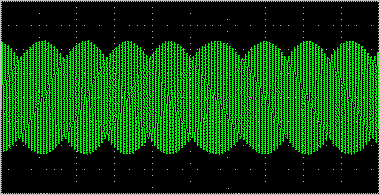Home > Glossary > Sample Rate# What is Sample Rate## Brief Introduction of Sample Rate

The sampling rate, sample rate, or sampling frequency defines the number of samples per second (or per other unit) taken from a continuous signal to make a discrete signal. For time-domain signals, the unit for sampling rate is hertz. The inverse of the sampling frequency is the sampling period or sampling interval, which is the time between samples.
Sample rate is usually noted in Sa/s (non-SI) and expanded as kSa/s, MSa/s, etc. The common notation for sampling frequency is fs which stands for frequency (subscript) sampled.

## Sampling Theorem

The Nyquist–Shannon sampling theorem states that perfect reconstruction of a signal is possible when the sampling frequency is greater than twice the maximum frequency of the signal being sampled, or equivalently, when the Nyquist frequency (half the sample rate) exceeds the highest frequency of the signal being sampled. If lower sampling rates are used, the original signal's information may not be completely recoverable from the sampled signal.

For example, if a signal has an upper band limit of 100 Hz, a sampling frequency greater than 200 Hz will avoid aliasing and allow theoretically perfect reconstruction.

*Oversampling

In some cases, it is desirable to have a sampling frequency more than twice the desired system bandwidth so that a digital filter can be used in exchange for a weaker analog anti-aliasing filter. This process is known as oversampling.

*Undersampling

Conversely, one may sample below the Nyquist rate. For a baseband signal (one that has components from 0 to the band limit), this introduces aliasing, but for a passband signal (one that does not have low frequency components), there are no low frequency signals for the aliases of high frequency signals to collide with, and thus one can sample a high frequency (but narrow bandwidth) signal at a much lower sample rate than the Nyquist rate.

## Related Software

Video Converter Factory Pro; it is an all in one and powerful video converter produced by WonderFox Soft, it is powerful fast and reliable and can help you convert both video and audio files, its rich parameter settings like setting the display resolution, bit rate, frame rate of your video files and sample rate, channels, bit rate, encoder and the volume of your audio files will surely help you get higher definition videos and good quality audios that you really need.

Video Converter Factory Pro, a really all in one video converter!

## Related Source